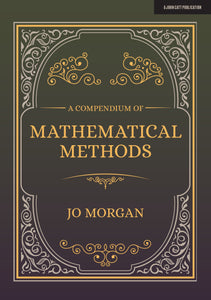# A Compendium Of Mathematical Methods

Regular price
\$24.95
Sale price
\$24.95
Unit price
per

How many different methods do you know to solve simultaneous equations? To multiply decimals? To find the nth term of a sequence?

A Compendium of Mathematical Methods brings together over one hundred different approaches from classrooms all over the world, giving curious mathematicians the opportunity to explore fascinating methods that they've never before encountered.

If you teach mathematics to any age group in any country, you are guaranteed to learn lots of new things from this delightful book. It will deepen your subject knowledge and enhance your teaching, whatever your existing level of expertise. It will inspire you to explore new approaches with your pupils and provide valuable guidance on explanations and misconceptions.

Woven throughout this compendium are extracts from antiquated maths textbooks. We glimpse into the past to see what has changed, and we marvel at the curiosities we encounter.

The following topics are featured: Subtraction; Multiplication; Decimal multiplication; Division; Fraction division; Highest common factor and lowest common multiple; Reverse percentages; Two-step linear equations; Linear simultaneous equations; Expanding double brackets; Factorising non-monic quadratics; Linear sequences; Quadratic sequences; Equations of linear graphs; Quadratic inequalities; Vertex of a quadratic; Simplifying surds; Angles in polygons; Polynomial division

Review

'This is a stunning book. A mathematical voyage across continents and through time. As a mathematician it makes me think harder about methods and procedures I take for granted. As a teacher it arms me with tools to help my students better understand concepts and spark scintillating conversations. And as an author... well, quite frankly, it makes me jealous. Congratulations Jo, this is wonderful.

-Craig Barton, Maths teacher, Author of How I wish I'd taught maths

In this book Jo combines her passion for good teaching and pedagogy, a expert magpie's eye for mathematical tidbits, and an enviable collection of historical mathematical books. In doing so she has created both a highly useful reference book for maths teachers, and a delightful set of curiosities just to enjoy dipping in to. I can see this book becoming an invaluable resource in school maths departments, and a great starting point for discussions which will keep even the more mundane techniques and methods of the subject feeling fresh and interesting. Even the most seasoned maths teacher is likely to discover something new in these pages, and I hope they will get as excited to share these things with their students as Jo is to share them with us. The scope is broad, and ranges from basic arithmetic methods, through equations of linear graphs, up to polynomial division and solving systems of equations using Gauss-Jordan elimination. Teachers, students, and recreational mathematicians will find plenty of interest and intrigue in this well researched and extremely useful collection.

-Ben Sparks (Mathematician, musician and teacher. Speaker, tutor and co-ordinator for the Advanced Maths Support Programme based at the University of Bath. Maths enrichment speaker )

A Compendium of Mathematical Methods is an impressive accessible collection of methods for teaching and explaining a multitude of mathematical topics. Each method is coherently explained through worked examples with Jo sharing her expert knowledge on the validity and history of methods. The result is a compelling practical resource for anyone involved in maths education – one that I’ll be referring to over and over.

-Emma McCrea (Author of Making Every Maths Lesson Count, Senior Lecturer at The University of Brighton, former Advanced Skills Teacher and Head of Maths)